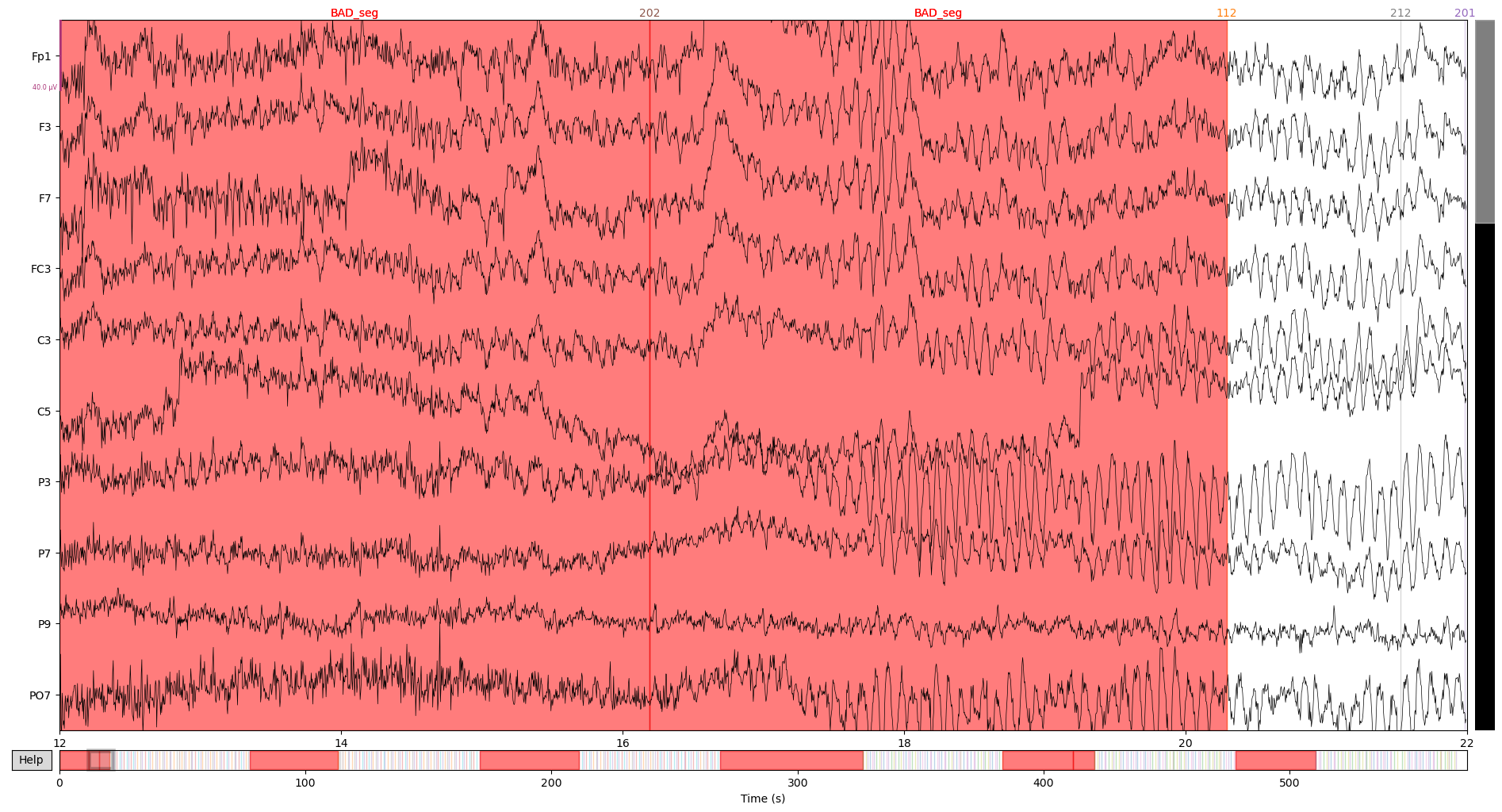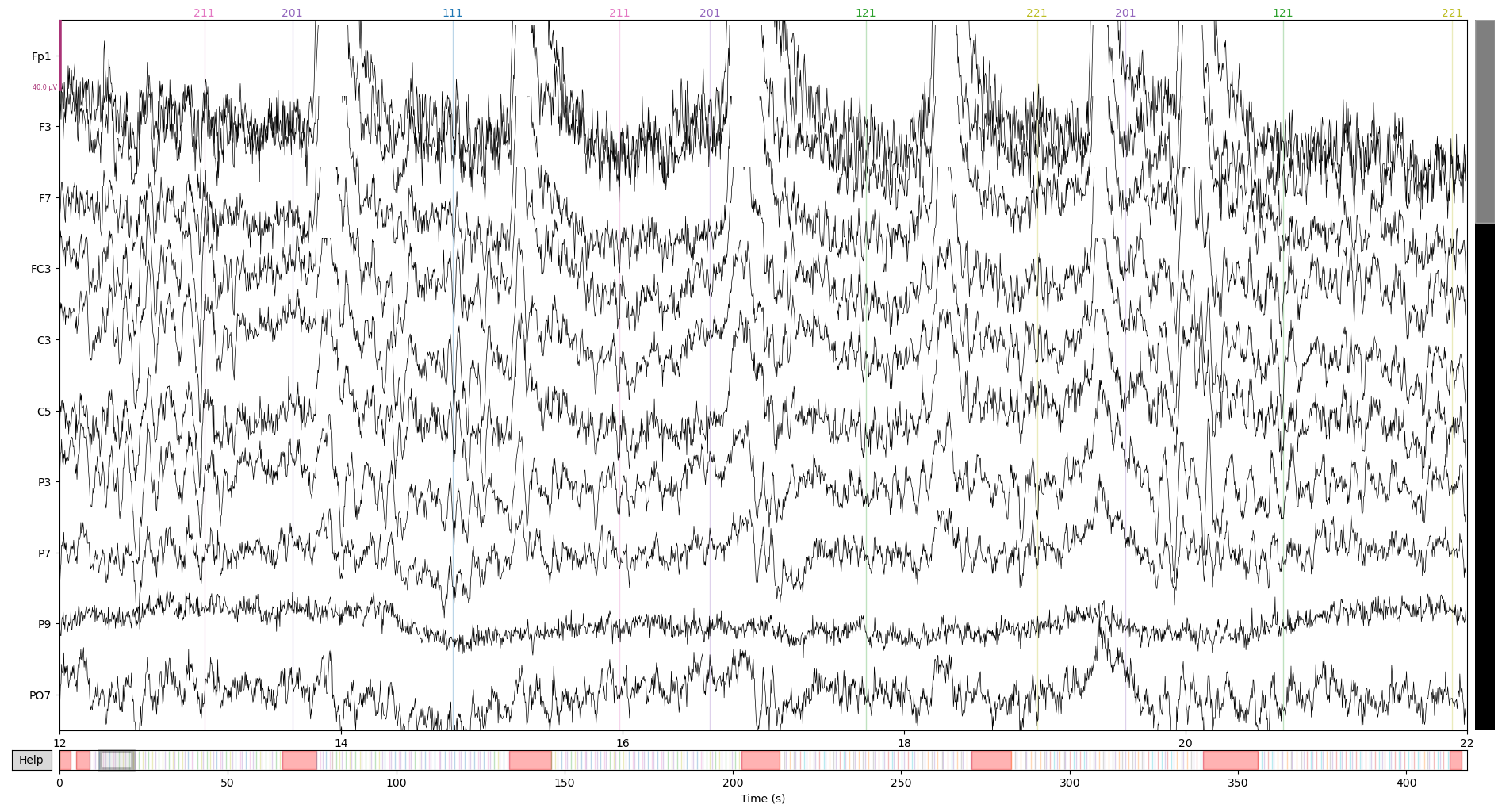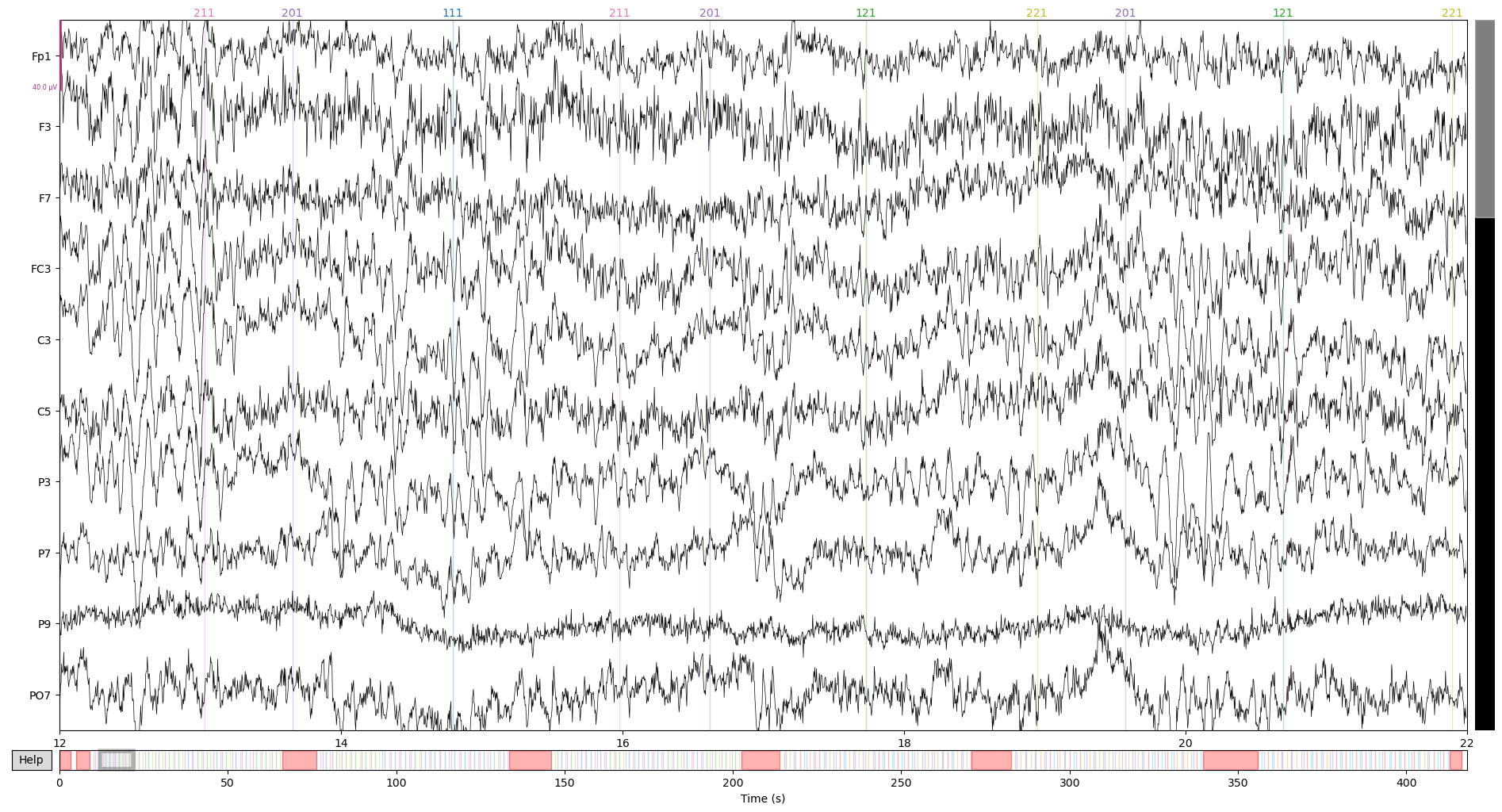# ERP Core N400 in MNE-Python: part II

This is the second part of adapting the ERP CORE N400 pipeline to MNE-Python. In the first part we did the first five steps (load, reference, downsample, montage and filter). Here, we will do artifact rejection based on Independent Component Analysis (ICA). As before, I show the steps for a single participant and wrap it in a loop at the end of the post.

### Import libraries and load data

# set working directory
import os
os.chdir('D:\\EEGdata\\Erp CORE\\N400')

import mne
# import some methods directly so we can call them by name

import numpy as np
import pandas as pd

# graphics definitions for jupyter lab
import matplotlib.pyplot as plt
%matplotlib qt
%gui qt


Load the data from where we left off in the last post:

raw = mne.io.read_raw("D:\\EEGdata\\Erp CORE\\N400\\mne\\raw\\N400_ERP_CORE_1-raw.fif", preload = True)


### ERP CORE script 2: ICA prep

The first thing to do is to mark intervals between experimental events with 2 or more seconds as bad segments. These segments will be ignored in ICA. In this N400 experiment, those long durations between events are either resting moments or moments were the participant was not really paying attention to the task, so they are probably very noisy.

Here we will craft the annotations indicating bad segments by hand and then add them to the existing ones. Each annotation is defined by a onset, a duration and a descripition (“BAD_seg” in our case). MNE automatically identifies annotations starting with “bad” in several functions to ignore the data marked.

bad_onsets = []
for n, ann in enumerate(raw.annotations):
# this condition is just so we don't break the loop at the end
if (n+1) < len(raw.annotations):
this_onset = ann['onset']
next_onset = raw.annotations[n+1]['onset']
t_between = next_onset - this_onset
# update the lists if time between stimuli >= 2secs
if t_between >= 2:


Sometimes, there is a long time before the first annotation appears. Our code above does not get these moments since the start is not an annotation in itself. Mark these kinds of segments, make a annotations structure with all new annotations and add them to the existing ones:

# add starting segment to bad ones if it is > 2s
if raw.annotations.onset >= 2:

# make annotations structure
# expand existing annotations


Lets see what it looks like, note the red segments in the timeline:

raw.plot(start = 12, n_channels = 10)### ERP Core Script 3: run ICA

We will run ICA for each subject. In the original pipeline, the authors add the weights obtained in ICA to the data object, in MNE we save a separate file with the fitted ica object.

The ERP CORE pipeline uses a tweaked version of ICA called binICA. I haven’t been able to delve into it’s code to fully replicate it with MNE. I refer the interested reader to the wiki page.

I have to say these data gave me kind of a hard time when I applied ICA to it. The default algorithm fastICA does not converge for several participants. So I ended up using the extended infomax which does not show convergence problems (I later found out binICA also uses the infomax algorithm). However, for many participants a HEOG component does not show (VEOG components are fine). I think part of the difficulty is because the ERP CORE experiment is not that long, and ICA can be quite data hungry.

In MNE, we do ICA in four major steps that revolve around an ica object that has all the ICA-related information and methods (parameters, fit, components to exclude etc.):

1. set parameters in a ica instance
2. fit the instance to the data with the object’s function fit
3. select components for exclusion in interactive visualizations
4. transform the data with the ica.apply method
# import the ICA class
from mne.preprocessing import ICA

# list of eog channels
eog_chans = ['VEOG_lower', 'HEOG_right', 'HEOG_left', 'HEOG', 'VEOG']
# list of channels to use in ica, at this point simply making a list with all channels
ica_chans = raw.info['ch_names'].copy()
# remove eog channels from list
for chan in eog_chans: ica_chans.remove(chan)

subj = 1
#load data to apply ica to
raw_path = "D:\\EEGdata\\Erp CORE\\N400\\mne\\raw\\N400_ERP_CORE_{}-raw.fif".format(subj)
# create ica instance with defined parameters
ica = ICA(.95, # number of components to extract. Here we use .95 meaning...
# ...'as many as necessary to account for 95% of data variance...
# in a preliminary PCA'
max_iter= 1000, # max iterations allowed for the algorithm
random_state=1, # this is just so you can replicate my results
method = 'infomax', # algorithm used
fit_params=dict(extended = True) # algorithm-specific parameters ...
)                               #...(in this case 'use extended infomax')

# fit ica with the parameters above to the data
ica.fit(raw, # data
picks = ica_chans, # channels to use
reject = dict(eeg = 300e-6) # threshold to ignore parts of the signal
)
# save ica object
ica_path = "D:\\EEGdata\\Erp CORE\\N400\\mne\\raw\\N400_ERP_CORE_{}-ica.fif".format(subj)
ica.save(ica_path)


Now we select the components to be excluded for each participant. The code below loads the ica object and the raw data and plots the topomap and source figures. The figures are interactive, if we click a component it is selected for exclusion. I should also mention that you can check more datailed information about each component with the function ica.plot_properties() (not shown here).

from mne.preprocessing import read_ica
subj = 2
ica_path = "D:\\EEGdata\\Erp CORE\\N400\\mne\\raw\\N400_ERP_CORE_{}-ica.fif".format(subj)
raw_path = "D:\\EEGdata\\Erp CORE\\N400\\mne\\raw\\N400_ERP_CORE_{}-raw.fif".format(subj)

ica.plot_sources(raw)

ica.plot_components()In this case, checking both figures, we select components one (VEOG artifacts) and two (HEOG artifacts) as bad components. After selecting, save the ica object with this new information.

ica.save(ica_path)


### ERP Core Script 4: remove ICA components

As in the ERP CORE pipeline, we remove the selected ICA components from the data and create a corrected HEOG channel. We will save this corrected data in a new file of raw data.

subj = 2
raw_path = "D:\\EEGdata\\Erp CORE\\N400\\mne\\raw\\N400_ERP_CORE_{}-raw.fif".format(subj)
ica_path =  "D:\\EEGdata\\Erp CORE\\N400\\mne\\raw\\N400_ERP_CORE_{}-ica.fif".format(subj)

# remove ica components from raw
raw_correct = ica.apply(raw)

#Create HEOG channel...
heog_ica_info = mne.create_info(['HEOG_ICA'], 256, "eog")
heog_ica_data = raw_correct['HEOG_left']-raw_correct['HEOG_right']
heog_ica_raw = mne.io.RawArray(heog_ica_data, heog_ica_info)

raw_correct_path = "D:\\EEGdata\\Erp CORE\\N400\\mne\\raw_corrected\\N400_ERP_CORE_{}_correct-raw.fif".format(subj)
raw_correct.save(raw_correct_path, overwrite=True)


Before we wrap things up, let’s inspect the “before and after” of the data to see ICA’s magic working. Before ICA:

subj = 3
raw_path = "D:\\EEGdata\\Erp CORE\\N400\\mne\\raw\\N400_ERP_CORE_{}-raw.fif".format(subj)

raw.plot(start = 12, n_channels = 10)After ICA:

raw_correct_path = "D:\\EEGdata\\Erp CORE\\N400\\mne\\raw_corrected\\N400_ERP_CORE_{}_correct-raw.fif".format(subj)

raw_corrected.plot(start = 12, n_channels = 10)On the first image, see that blinking artfact right on top of the 14s timepoint? ICA got rid of it for the most part, pretty neat!

For the next post, we will finish preprocessing and partition the data into epochs.

Lastly, the loops to fit ICA for all participants.

# import the ICA class
from mne.preprocessing import ICA

# list of eog channels
eog_chans = ['VEOG_lower', 'HEOG_right', 'HEOG_left', 'HEOG', 'VEOG']
# list of channels to use in ica, at this point simply making a list with all channels
ica_chans = raw.info['ch_names'].copy()
# remove eog channels from list
for chan in eog_chans: bipolar_chans.remove(chan)

for subj in range(1, 41):
#load data to apply ica to
raw_path = "D:\\EEGdata\\Erp CORE\\N400\\mne\\raw\\N400_ERP_CORE_{}-raw.fif".format(subj)
# create ica instance with defined parameters
ica = ICA(.95, # number of components to extract. Here we use .95 meaning...
# ...'as many as necessary to account for 95% of data variance...
# in a preliminary PCA'
max_iter= 1000, # max iterations allowed for the algorithm
random_state=1, # this is just so you can replicate my results
method = 'infomax', # algorithm used
fit_params=dict(extended = True) # algorithm-specific parameters ...
)                               #...(in this case 'use extended infomax')

# fit ica with the parameters above to the data
ica.fit(raw, # data
picks = ica_chans, # channels to use
reject = dict(eeg = 300e-6) # threshold to ignore parts of the signal
)
# save ica object
ica_path = "D:\\EEGdata\\Erp CORE\\N400\\mne\\raw\\N400_ERP_CORE_{}-ica.fif".format(subj)
ica.save(ica_path)

# After this, mark bad components for each participant as show above in the post
#Then apply the correction:

for subj in range(1, 41):
raw_path = "D:\\EEGdata\\Erp CORE\\N400\\mne\\raw\\N400_ERP_CORE_{}-raw.fif".format(subj)
ica_path =  "D:\\EEGdata\\Erp CORE\\N400\\mne\\raw\\N400_ERP_CORE_{}-ica.fif".format(subj)

# remove ica components from raw
raw_correct = ica.apply(raw)

#Create HEOG channel...
heog_ica_info = mne.create_info(['HEOG_ICA'], 256, "eog")
heog_ica_data = raw_correct['HEOG_left']-raw_correct['HEOG_right']
heog_ica_raw = mne.io.RawArray(heog_ica_data, heog_ica_info)